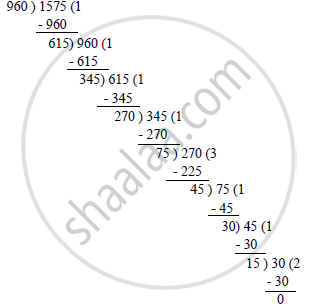# Using Euclid’S Algorithm, Find the Hcf Of 960 and 1575 . - Mathematics

Using Euclid’s algorithm, find the HCF of  960 and 1575 .

#### SolutionOn applying Euclid’s algorithm, i.e. dividing 1575 by 960, we get:
Quotient = 1, Remainder = 615
∴ 1575 = 960 × 1 + 615
Again on applying Euclid’s algorithm, i.e. dividing 960 by 615, we get:
Quotient = 1, Remainder = 345
∴ 960 = 615 × 1 + 345
Again on applying Euclid’s algorithm, i.e. dividing 615 by 345, we get:
Quotient = 1, Remainder = 270
∴ 615 = 345 × 1 + 270
Again on applying Euclid’s algorithm, i.e. dividing 345 by 270, we get:
Quotient = 1, Remainder = 75
∴ 345 = 270 × 1 + 75
Again on applying Euclid’s algorithm, i.e. dividing 270 by 75, we get:
Quotient = 3, Remainder = 45
∴ 270 = 75 × 3 + 45
Again on applying Euclid’s algorithm, i.e. dividing 75 by 45, we get:
Quotient = 1, Remainder = 30
∴ 75 = 45 × 1 + 30
Again on applying Euclid’s algorithm, i.e. dividing 45 by 30, we get:
Quotient = 1, Remainder = 15
∴ 45 = 30 × 1 + 15
Again on applying Euclid’s algorithm, i.e. dividing 30 by 15, we get:

Quotient = 2, Remainder = 0
∴ 30 = 15 × 2 + 0
Hence, the HCF of 960 and 1575 is 15.

Concept: Euclid’s Division Lemma
Is there an error in this question or solution?